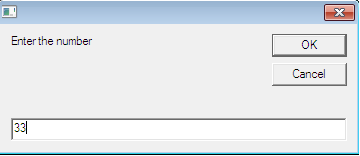## Saturday, 29 March 2014

### Functions in VBScript

Functions are used to perform the specific task.Functions are used to increase the reusability of the code. Functions are similar to the procedures except one difference that functions can return value.

## Simple example of the function

Suppose you want to find the sum of 2 numbers. Without functions, you will write below code.

```a = 10
b = 20
c = a+ b
Msgbox c```

To add another 2 different numbers, you will use below code

```a = 33
b = 65
c = a+ b
Msgbox c```

In above examples, we have to add 2 numbers. So the operation is same (Repeating) So we can write the function which will take 2 parameters as shown below.

```function sum(byref a, byref b)

sum = cint(a) + cint(b)

end function```

## Calling functions in VBScript

To call the procedures we can use below statements.

```c = sum(10,20)
msgbox c
c =  sum(33,65)
msgbox c```

Thus we can call functions many times in the code. This reduces the lines of code  as well as maintainability of the code.

## Passing arguments to the function

We can pass the arguments to the function by 2 ways.
1. Pass by reference
2. Pass by value
Pass by reference

By default, values are passed to the function by reference. When we pass the values by reference the changes made to the variables in the called function are reflected in the calling function/procedure.

```a = 10
c = findsqr(a)
msgbox a  'prints 100
msgbox c  'prints 100

function findsqr(byref a)

a = a*a

findsqr = a

end function```

Pass by value

When we pass the values by value, the changes made to the variables in the called function are not reflected in the calling procedure or function.

```a = 10
c = findsqr(a)
msgbox a  'prints 10
msgbox c  'prints 100

function findsqr(byval a)

a = a*a

findsqr = a

end function```

## Other built-in functions in VBScript

1. msgbox
2. inputbox
3. eval
4. execute
msgbox function is used to show message to the user.
inputbox function is used to read the value from the user.

```a = inputbox("Enter the number")
msgbox a 'prints 33```Inputbox function in vbscript
Eval and execute functions are used to execute any valid vbscript expression. Difference between 2 functions is that the operator = is handled in different ways.

```Eval always uses = as a comparison operator.
Execute always uses = as a assignment operator.```

`Example -`
```a=20
msgbox eval("a=10")  'will print false
msgbox a

a=20
execute("a=10")
msgbox a    'will print 10```